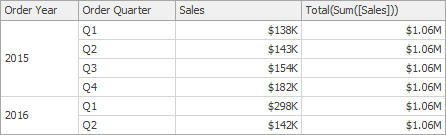# Window Calculation Functions

This topic contains a description of window functions that can be used to specify measure expressions.

Index()

Returns the index of the current row in the window.

`Index()`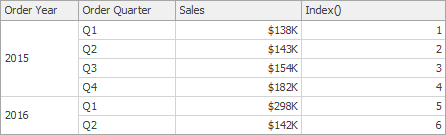Size()

Returns the number of rows in the window.

`Size()`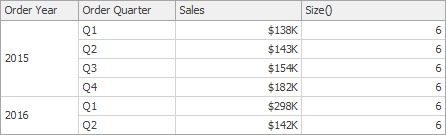Lookup(SummaryExpression, Position)

Returns the value of the expression in a target position specified as a relative offset from the current position.

`Lookup(Sum([Sales]), 3)`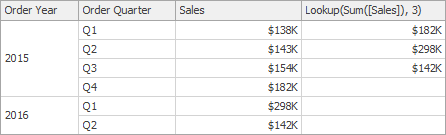Last()

Returns the number of rows from the current row to the last row in the window.

`Last()`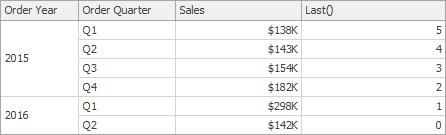First()

Returns the number of rows from the current row to the first row in the window.

`First()`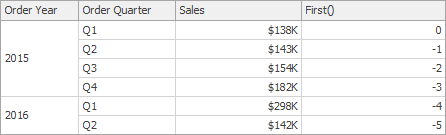RankCompetition(SummaryExpression, [ ‘asc’ | ‘desc’ ])

Returns the standard competition rank for the current row in the window.

`RankCompetition(Sum([Sales]), 'asc')`

RankDense(SummaryExpression, [ ‘asc’ | ‘desc’ ])

Returns the dense rank for the current row in the window.

`RankDense(Sum([Sales]), 'asc')`

RankUnique(SummaryExpression, [ ‘asc’ | ‘desc’ ])

Returns the unique rank for the current row in the window.

`RankUnique(Sum([Sales]), 'asc')` RankModified(SummaryExpression, [ ‘asc’ | ‘desc’ ]) Returns the modified competition rank for the current row in the window.

`RankModified(Sum([Sales]), 'asc')` RankPercentile(SummaryExpression, [ ‘asc’ | ‘desc’ ]) Returns the percentile rank for the current row in the window.

`RankPercentile(Sum([Sales]), 'desc')`

RunningAvg(SummaryExpression)

Returns the running average of the specified expression from the first row in the window to the current row.

`RunningAvg(Sum([Sales]))`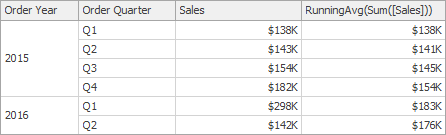RunningAggregate(SummaryExpression, CustomAggregateName)

Returns the cumulative result of the custom function applied to specified expression from the first row in the window to the current row.

`RunningAggregate(Min([City]), 'StringConcat')`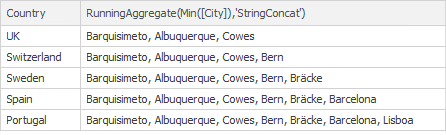RunningCount(SummaryExpression)

Returns the running count of the specified expression from the first row in the window to the current row.

`RunningCount(Sum([Sales]))`

RunningMax(SummaryExpression)

Returns the running maximum of the specified expression from the first row in the window to the current row.

`RunningMax(Sum([Sales]))`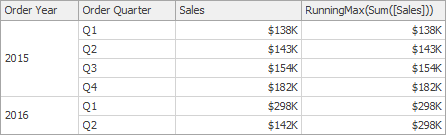RunningMin(SummaryExpression)

Returns the running minimum of the specified expression from the first row in the window to the current row.

`RunningMin(Sum([Sales]))`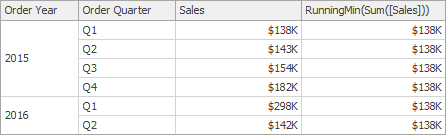RunningSum(SummaryExpression)

Returns the running sum of the specified expression from the first row in the window to the current row.

`RunningSum(Sum([Sales]))`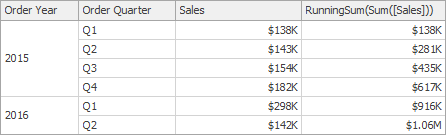WindowAggregate(SummaryExpression, StartOffset, EndOffset, CustomAggregateName)

Returns the result of the custom function applied to the specified expression in the window.

`WindowAggregate(Min([CompanyName]), -1, 1, 'StringConcat')`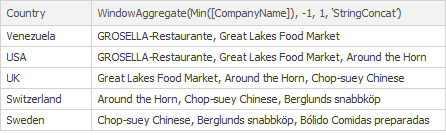WindowAvg(SummaryExpression, StartOffset, EndOffset)

Returns the average of the expression within the window, which is defined using offsets from the current row.

`WindowAvg(Sum([Sales]), First(), Last())`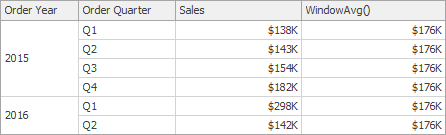WindowCount(SummaryExpression, StartOffset, EndOffset)

Returns the count of the expression within the window.

`WindowCount(Sum([Sales]), First()+2, Last())`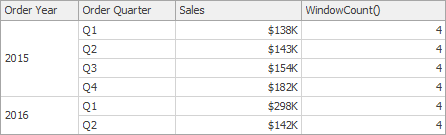WindowCountDistinct(SummaryExpression, StartOffset, EndOffset)

Returns the distinct count of the expression within the window.

`WindowCountDistinct(Sum([Sales]), First(), Last())`

WindowFirstValue(SummaryExpression, StartOffset, EndOffset)

Returns the first value of the expression within the window.

`WindowFirstValue((Sum([Sales]), First(), Last())`

WindowMax(SummaryExpression, StartOffset, EndOffset)

Returns the maximum of the expression within the window.

`WindowMax(Sum([Sales]), First(), Last())`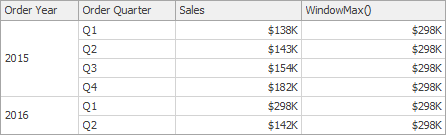WindowMin(SummaryExpression, StartOffset, EndOffset)

Returns the minimum of the expression within the window.

`WindowMin(Sum([Sales]), First(), Last())`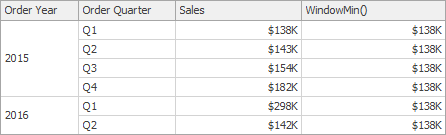WindowMode(SummaryExpression, StartOffset, EndOffset)

Returns the statistical mode in the window (the element that occurs most often in the values calculated with the specified expression within the window).

`WindowMode(Sum([Sales]), First(), Last())`

WindowMedian(SummaryExpression, StartOffset, EndOffset)

Returns the median of the expression within the window.

`WindowMedian(Sum([Sales]), First(), Last())`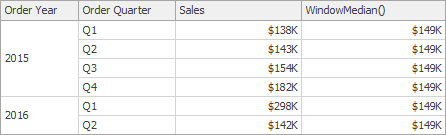WindowSum(SummaryExpression, StartOffset, EndOffset)

Returns the sum of the expression within the window.

`WindowSum(Sum([Sales]), First()+2, Last())`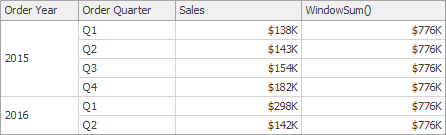WindowVar(SummaryExpression, StartOffset, EndOffset)

Returns the variance of the expression within the window.

`WindowVar(Sum([Sales]), First(), Last())`

WindowVarp(SummaryExpression, StartOffset, EndOffset)

Returns the biased variance of the expression within the window.

`WindowVarp(Sum([Sales]), First(), Last())`

WindowStdDev(SummaryExpression, StartOffset, EndOffset)

Returns the sample standard deviation of the expression within the window.

`WindowStdDev(Sum([Sales]), First(), Last())`

WindowStdDevp(SummaryExpression, StartOffset, EndOffset)

Returns the biased standard deviation of the expression within the window.

`WindowStdDevp(Sum([Sales]), First(), Last())` Total(SummaryExpression)

Returns the total for the specified expression in a calculation window. Note that the Total function calculates the total based on values from the underlying data source.

`Total(Sum([Sales]))`